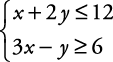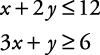## Linear Inequalities: Solutions Using Graphing with Two Variables

##### Example 1

Graph the solution to this system of inequalities.To graph the solution to a system of inequalities, follow this procedure:

1. Graph each sentence on the same set of axes.

2. See where the shading of the sentences overlaps.

The overlapping region is the solution to the system of inequalities. The solution to the system is the region with both shadings (see Figure 1).

The solution to the following is, therefore, as shown in Figure 2.